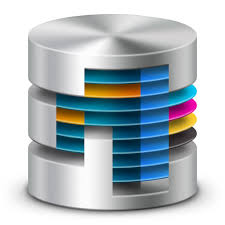# How to create scalar function of SQL Server in order to calculate GNRI?Pocket

Malnutrition in elder people increases the risk of death. Geriatric Nutrition Risk Index (GNRI) is the tool to detect malnutrition easily in hemodialysis patients, too. The definition is as following;$\displaystyle \mathrm{GNRI} = 14.89\times\mathrm{Albumin (g/dL)} + 41.7\times\frac{\mathrm{Body Weight}}{\mathrm{Ideal Body Weight}}$

where ideal body weight is given by multiplying 22 the square of height. But body weight should be replaced with ideal body weight if body weight is greater than ideal body weight. Then the second term is equal to 1.

Table is defined as following procedure. It is based on the survey list of the Japanese Society for Dialysis Therapy in 2013.

We need height, body weight and albumin in the table. Execute following procedure to create function.

Execute following query to calculate GNRI.

Pocket